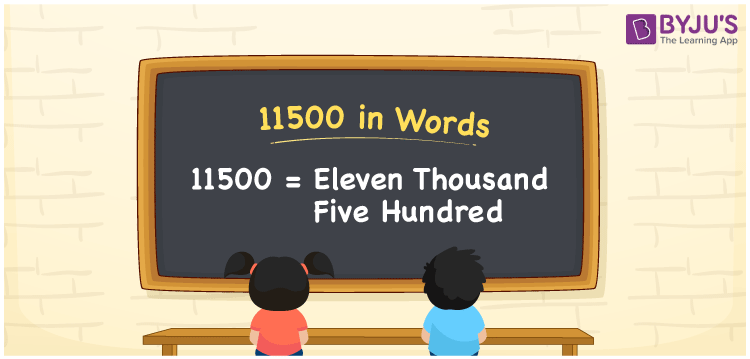# 11500 in words

11500 in words is written as Eleven thousand five hundred. 11500 represents the count or value. The article on Counting Numbers can give you an idea about count or counting. The number 11500 is used in expressions that relate to money, distance, length and many more.

In case of money, an example would look like this: “If I have to deposit 11500 Rupees in the bank without any issues then in the cheque I need to write the number 11500 in words correctly. And that would be “Eleven thousand five hundred” Only.

 11500 in words Eleven Thousand Five Hundred Eleven Thousand Five Hundred in Numbers 11500

## 11500 in English Words## How to Write 11500 in Words?

We can convert 11500 to words using a place value chart. This can be done as follows. The number 11500 has 5 digits, so let’s make a chart that shows the place value up to 5 digits.

 Ten thousands Thousands Hundreds Tens Ones 1 1 5 0 0

Thus, we can write the expanded form as:

1 × Ten thousand + 1 × Thousand + 5 × Hundred + 0 × Ten + 0 × One

= 1 × 10000 + 1 × 1000 + 5 × 100 + 0 × 10 + 0 × 1

= 11500

= Eleven thousand five hundred

11500 is the natural number that is succeeded by 11499 and preceded by 11501.

11500 in words – Eleven thousand five hundred

Is 11500 an odd number? – No.

Is 11500 an even number? – Yes

Is 11500 a perfect square number? – No

Is 11500 a perfect cube number? – No

Is 11500 a prime number? – No

Is 11500 a composite number? – Yes

## Frequently Asked Questions on 11500 in Words

### How to write 11500 in words?

11500 in English is written as “eleven thousand five hundred”.

### Is 11500 a prime number?

No, the number 11500 is not a prime number. Eleven thousand five hundred is a composite number. Because 11500 has more divisors than 1 and itself.

### Write eleven thousand five hundred in numbers.

Eleven thousand five hundred in numbers is 11500.